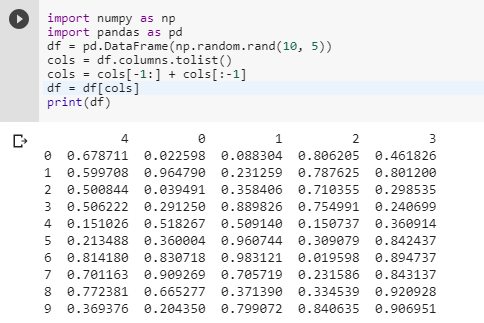2 views
in Python

I have the following DataFrame (df):

import numpy as np

import pandas as pd

df = pd.DataFrame(np.random.rand(10, 5))

I add more column(s) by assignment:

df['mean'] = df.mean(1)

How can I move the column mean to the front, i.e. set it as the first column leaving the order of the other columns untouched?

by (47.6k points)

The below-mentioned code will help you to get the desired result :

import numpy as np

import pandas as pd

df = pd.DataFrame(np.random.rand(10, 5))

cols = df.columns.tolist()

cols = cols[-1:] + cols[:-1]

df = df[cols]

print(df)